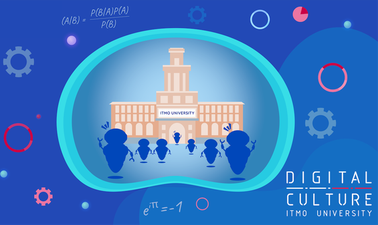Introduction to Machine Learning

Learn the essentials of machine learning and algorithms of statistical data analysis.This course is archived
Estimated 5 weeks
2–4 hours per week
Self-paced
Free

Want to learn how to analyze the huge amounts of data? In this course you will learn modern methods of machine learning to help you choose the right methods to analyze your data and interpret the results correctly.

This course is an introduction to machine learning. It will cover the modern methods of statistics and machine learning as well as mathematical prerequisites for them. We will discuss the methods used in classification and clustering problems. You will learn different regression methods.

Various examples and different software applications are considered in the course. You will get not only the theoretical prerequisites, but also practical hints on how to work with your data in MS Azure.

What you'll learn

Skip What you'll learn
• Introduction to machine learning and mathematical prerequisites
• Regression types (linear, polynomial, multi variable regression)
• Classification methods: Logistic regression, Naïve Bayes and K-nearest neighbours
• Clustering methods: hierarchical and k-means clustering

Syllabus

Skip Syllabus

Week 1: Introduction to machine learning and mathematical prerequisites. The concepts of machine and statistical learning are introduced. We discuss the main branches of ML such as supervised, unsupervised and reinforcement learning, give specific examples of problems to be solved by the described approaches. Besides, we show that ML is not as powerful as one can think. Finally, we remind you of some basic concepts of mathematics used in further lectures.

Week 2: Regression (linear, polynomial, multivariable regression). Regression problem is one of the main problems in supervised learning. We start with the heuristic approach trying to solve a very practical problem and come to rigorous mathematical construction of the simple linear regression model. We go further and describe statistical properties of the model: confidence intervals for the model's parameters, hypothesis testing of linear dependence. Finally, we come to a so-called multivariable linear and polynomial regressions and show some examples and applications.

Week 3: Logistic regression. The second branch of supervised learning is a classification problem. We deal with a two-class logistic regression and emphasise that it is not a regression at all. Then why is it called so? It's construction is closely connected with linear regression described in the 2nd lecture. We remind you a maximum likelihood estimation method and its applications to logistic regression. Finally, we discuss some applications of the logistic regression to a football game predictions and describe ROC analysis or a quality testing approach for the described model.

Week 4: Naïve Bayes and K-nearest neighbors. In this lecture we continue with classification problem. We introduce a so-called naive Bayes approach to classification widely used in e-mail spam recognition until 2010. Then we come to a multi-class classification using K-nearest neighbors method. What are the metrics that we will use? How does a particular metric influence the result? What is K and how do you choose it solving a particular problem? These are the questions that are rigorously discussed in the lecture.

Week 5: Clustering methods: hierarchical and k-means clustering. Clusterization problem is at the heart of unsupervised learning. We have a lot of data and nothing else: we don't know the amount of classes, similarities in objects, we know almost nothing. We show how to establish some order in the given chaotic data using hierarchical clustering method and k-means approach. How to establish the initial clusters, what metric to choose, what actually means "close and far" objects? These questions are discussed in the lecture.